RS Aggarwal Class 8 Solutions Chapter 21 - Data Handling

RS Aggarwal Class 8 Chapter 21 - Data Handling Solutions Free PDF

RS Aggarwal class 8 solutions chapter 21 data handling is provided here. In this chapter, the students of class 8 will learn how to organize and group data. After learning the basics students will learn how to draw a bar graph and a pie chart with the help of given data. Practice the concepts of data handling by solving the questions from RS Aggarwal for class 8. RS Aggarwal book has step by step methods of how to approach a given problem.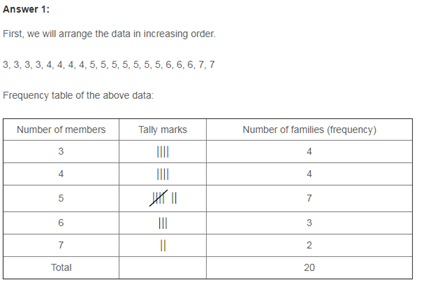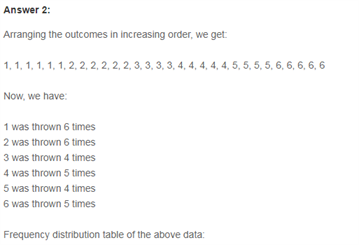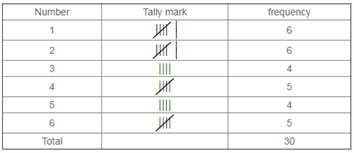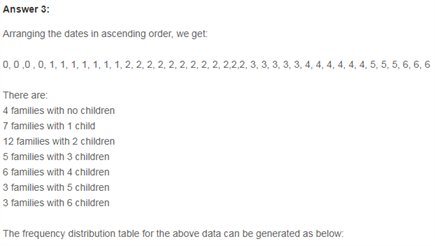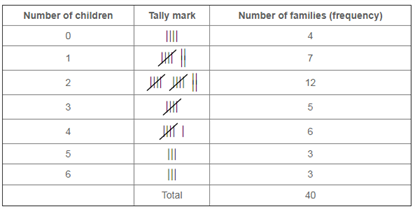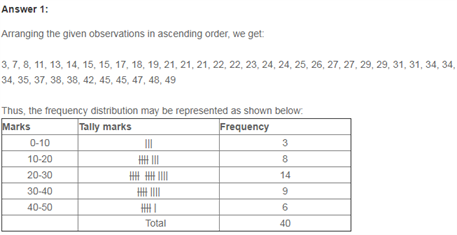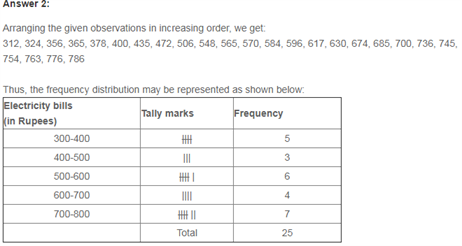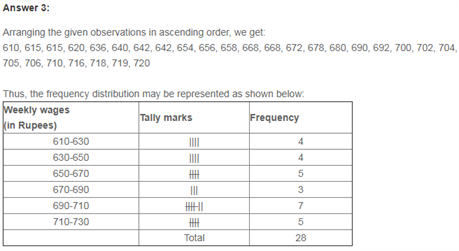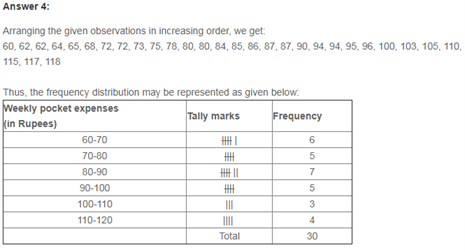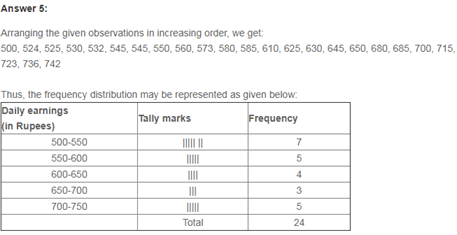Practise This Question

Choose the statements which state laws of reflection correctly.

Statement 1: Angle of incidence is greater than the angle of reflection.

Statement 2: Angle of incidence is less than the angle of reflection.

Statement 3: Angle of incidence is equal to the angle of reflection.

Statement 4: The incident ray, the normal at point of incidence and the reflected ray all lie in the same plane.